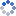加载中…菜鸟的成长
• 博客等级：• 博客积分：0
• 博客访问：597,552
• 关注人气：226
• 获赠金笔：0支
• 赠出金笔：0支
• 荣誉徽章：

## 利用R语言绘制韦恩图

(2012-12-16 21:10:22)

### 杂谈

library(VennDiagram)

A = 1:150
B = c(121:170,300:320)
C = c(20:40,141:200)
Length_A<-length(A)
Length_B<-length(B)
Length_C<-length(C)
Length_AB<-length(intersect(A,B))
Length_BC<-length(intersect(B,C))
Length_AC<-length(intersect(A,C))
Length_ABC<-length(intersect(intersect(A,B),C))

T<-venn.diagram(list(A=A,B=B),filename=NULL
,lwd=1,lty=2
,col=c('red','green'),fill=c('red','green')
,cat.col=c('red','green')
,rotation.degree=90)
grid.draw(T)

T<-venn.diagram(list(A=A,B=B,C=C),filename=NULL
,lwd=1,lty=2,col=c('red','green','blue')
,fill=c('red','green','blue')
,cat.col=c('red','green','blue')
,reverse=TRUE)
grid.draw(T)

draw.pairwise.venn(area1=Length_A,area2=Length_B,cross.area=Length_AB
,category=c('A','B'),lwd=rep(1,1),lty=rep(2,2)
,col=c('red','green'),fill=c('red','green')
,cat.col=c('red','green')
,rotation.degree=90)

draw.triple.venn(area1=Length_A, area2=Length_B, area3=Length_C
,n12=Length_AB, n23=Length_BC, n13=Length_AC, n123=Length_ABC
,category = c('A','B','C')
,col=c('red','green','blue'),fill=c('red','green','blue')
,cat.col=c('red','green','blue')
,reverse = FALSE)
draw.pairwise.venn类似，area1、area2、area3分别指第一个、第二个、第三个集合的大小。n12表示第一个与第二个集合的交集大小，n23、n13也是类似，n123指三个集合的交集大小。reverse则指是否对图形进行反转。

0